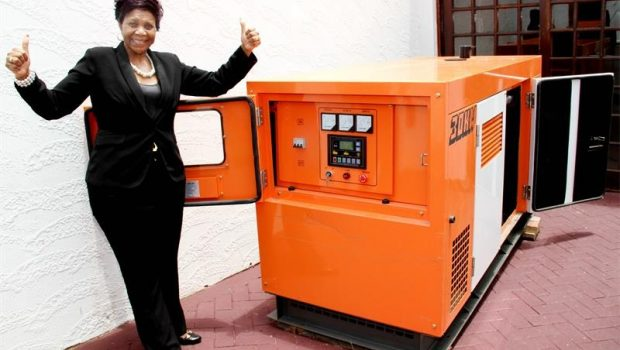# How To Calculate The Electricity Consumption To Buy A Generator For A House?The electricgenerators are useful devices, which come in different models and with various capacities. That is why when selecting the most suitable for a house, we must take into account several criteria. The maincriterion is the electricity consumption generated in the home, which must be covered by the generator to be purchased.

In this article we will describe in detail each of the aspects to consider in the purchase of an electric generator, in order to calculate the electrical consumption of appliances, tools and other equipment that work in a home, also do refer to electrician Rhodeisland for more advise.

# Physical Units And Their Conversions

We must know very well the physical units related to the electrical consumption of the devices, in order to perform the calculations correctly.

First let’s review the terms of:

• Amperage (A): is the electric current that requires a device to operate.
• Voltage (V): is the electrical voltage that power plants supply to companies and homes. This may vary by country, and ranges from 100V to 440V.
• Watt (W): is the unit of electrical power.

To calculate electrical power (W), that is, the electrical consumption of a device, the electric current (A) is multiplied by the voltage (V): A * V = W

Generally, the power of the devices is expressed in watts, but When power is high, multiples such as kilowatt (kW) or megawatt (MW) are often used.

• 1 kW = 1,000 W
• 1 MW = 1,000,000 W

Kilovoltiamperio (kVA): is a multiple of the voltiamperio (VA), that is the unit of the apparent power and the complex power of an electrical device.

The kVA is the unit used to indicate the power of an electric generator.

# How to calculate the electrical power of a device?

To calculate the electrical consumption of our entire house, we must begin by knowing how the electrical use of a single device or electrical appliance is calculated. Taking into account the definitions and relationship of the physical units already mentioned, we will turn to an example:Suppose we have a laptop with 1A to 220V, applying the formula to calculate the electrical power, we will obtain the consumption of this device: 1A x 220V = 220W

Usually, the electrical equipment has a label or sheet where its electrical power is indicated, so we would not have to calculate it. However, there is also an equipment that only indicates its amperage (A) and its voltage (V), in which case we must apply the indicated formula to know the electrical power of said equipment.In case one or more of the devices that we have in our home, have a high electrical power, we will get that this is expressed in kW or MG. In either case, we would only have to convert these values ​​to W, using the values ​​of equivalence.

For example, suppose we have a powerful tool whose label indicates that its power is 350 kW. To convert this value to watts, we only have to multiply it by the equivalence: 350 kW * 1000 = 350.000 W